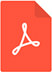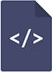# Petro physical analysis of wells in explorer field, offshore Niger delta, Nigeria using well log and core data

International Journal of Petrochemical Science & Engineering
Lurogho Sayoleyi,1 Ideozu UIdeozu,2 Abe J Sunday3PDF Full Text

# Abstract

Petro physical Analysis of Wells in Explorer Field Offshore Niger Delta, Nigeria is the focus of this research. Data used in this research well logs and core data that were integrated to evaluate the reservoir characteristics of Explorer Field. The objectives of this research is to determine lithology, shale volume (Vsh), porosity(Φ), permeability (K), fluid saturation and cross plotting of petro physical and core values at specific intervals to evaluate their level of correlation. Twelve hydrocarbon-bearing reservoirs from three wells were in this research. The average permeability value of the reservoirs is 20.0140md while porosity value ranges between 18%-39%. Fluid type defined in the reservoirs based on neutron/density log signature indicates water, oil and gas, low water saturation values ranging from 2.9% to 46% in Explorer wells indicate high hydrocarbon saturation. The Pearson Correlation Coefficient and Regression Equation gave a significant relationship between petro physical derived data and core data. Scatter plot of petro physical gamma ray values versus core gamma ray values gave an approximate linear relationship with correlation coefficient values of 0.6642, 0.9831 and 0.3261. Cross plots of well log determined density values and core density values revealed that there is a strong linear relationship between the two sets of data set with correlation coefficient values of 0.7581, 0.9872 and 0.3557, and the regression equation confirmed the relationship between the two data sets. In addition, the scatter plot of well log derived porosity/density values versus core porosity/ density values revealed a strong linear relationship between the two data sets with correlation coefficient values of 0.7608 and 0.9849; the regression equation confirmed this also. Cross plots of well log derived porosity/density values versus core porosity/density values in Well 3 gave very weak correlation coefficient values of 0.3261 and 0.3557 with a negative slope. The petro physical properties of the reservoirs in Explorer Well showed that they contain hydrocarbon in commercial quantity and the cross plot of the petro physical and core values showed direct relationship in most of the wells.

# Keywords

petro physics, correlation, regression, reservoirs, characterization, hydrocarbon and saturation, hydrocarbon saturation, sedimentology, structural geology